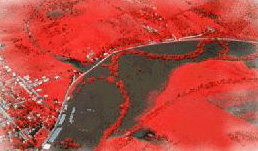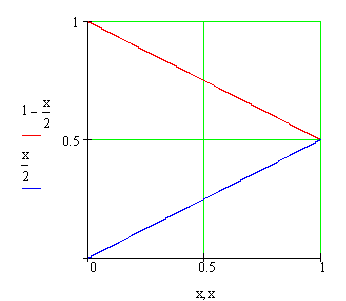Calculus on Demand at Dartmouth College Lecture 23 | Index | Lecture 25 Lecture 24## Resources

Math 3 Course Syllabus
Practice Exams

# Contents

In this lecture we discuss the Trapezoid Rule, a numerical technique for evaluating (approximately) a definite integral. We also discuss a formula for finding the area between two curves.

### Quick Question

What is the area between the two curves f(x) = 1 − x/2 (in red) and g(x) = x/2 (in blue)?### Outline

Outlines for
The Trapezoid Rule
Area Between Curves

### Textbook

The Trapezoid Rule and Simpson's Rule
Area Between Curves